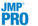Publication date: 07/30/2020

##Validation Method Options

The following methods are available for validation of the model fit.

Note: The only Validation Method allowed for Quantile Regression is None. The only Validation Methods allowed for the Maximum Likelihood Estimation Method are None and Validation Column. The only Validation Methods allowed for Cox Proportional Hazards are BIC, AICc, and None. The only Validation Methods allowed for the Dantzig Selector Estimation Method are BIC and AICc.

KFold

For each value of the tuning parameter, the following steps are conducted:

The observations are partitioned into k subsets, or folds.

In turn, each fold is used as a validation set. A model is fit to the observations not in the fold. The log-likelihood based on that model is calculated for the observations in the fold, providing a validation log-likelihood.

The mean of the validation log-likelihoods for the k folds is calculated. This value serves as a validation log-likelihood for the value of the tuning parameter.

The value of the tuning parameter that has the maximum validation log-likelihood is used to construct the final solution. To obtain the final model, all k models derived for the optimal value of the tuning parameter are fit to the entire data set. Of these, the model that has the highest validation log-likelihood is selected as the final model. The training set used for that final model is designated as the Training set and the holdout fold for that model is the Validation set. These are the Training and Validation sets used in plots and in the reported results for the final solution.

Holdback

Randomly selects the specified proportion of the data for a validation set, and uses the other portion of the data to fit the model. The final solution is the one that minimizes the negative log-likelihood for the validation set. This method is useful for large data sets.

Leave-One-Out

Performs leave-one-out cross validation. This is equivalent to KFold, with the number of folds equal to the number of rows. This option should not be used on moderate or large data sets. It can require long processing time for even a moderate number of observations. The Training and (one-row) Validation sets used in plots and in the reported results for the final solution are determined as is done for KFold validation.

BIC

Minimizes the Bayesian Information Criterion (BIC) over the solution path. See Likelihood, AICc, and BIC in the Statistical Details section.

AICc

Minimizes the corrected Akaike Information Criterion (AICc) over the solution path. AICc is the default setting for Validation Method. See Likelihood, AICc, and BIC in the Statistical Details section.

Note: The AICc is not defined when the number of parameters approaches or exceeds the sample size.

ERIC

Minimizes the Extended Regularized Information Criterion (ERIC) over the solution path. See Model Fit Detail. Available only for exponential family distributions and for the Lasso and adaptive Lasso estimation methods.

None

Does not use validation. Available only for the Maximum Likelihood Estimation Method and Quantile Regression.

Validation Column

Uses the column specified in the Fit Model window as having the Validation role. The final solution is the one that minimizes the negative log-likelihood for the validation set. This option is not available when the specified Estimation Method is Dantzig Selector or when the specified Distribution is Quantile Regression or Cox Proportional Hazards.